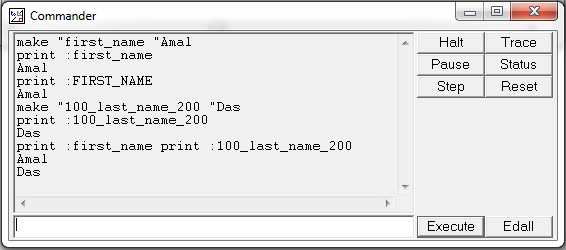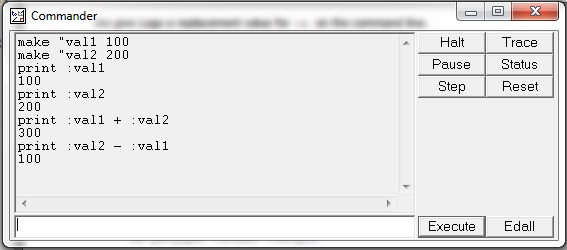# Logo - Variables

A variable is the name of a memory location which can contain a value. In a computer, each memory location has an integer address. Since it would be hard to remember the address of each location containing a value used by a program, computer scientists have found ways of giving these locations, symbolic names. Once a variable has a name, we can use and manipulate it.

Variables are given names which are strings of letters. A variable name can contain alphabets (case insensitive), digits and underscore. A variable name can be accessed in a computation using ‘:’ before it. Let us consider the following example in the screenshot.In the above example, we have defined two variables first_name, 100_last_name_200 and initialized them with values Amal and Das using the following statements −

• make “first_name “Amal
• make “100_last_name_200 “Das

Also, we printed these two variables with statements print :first_name and print :100_last_name_200.

The following example shows how to define numeric variables −Here, we have defined two numeric variables val1 and val2. We have also performed addition and subtraction using them.